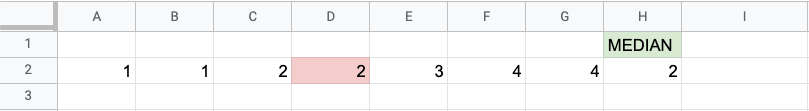## MEDIAN Function

The MEDIAN function is a premade function in Google Sheets, which returns the middle value in the data.

It is typed `=MEDIAN` and gets a list of cells:

=MEDIAN(value1, [value2, ...])

Note: The median is a type of average value, which describes where the center of the data is located. You can learn about median in our Statistics Median Tutorial.

Calculating the median manually requires that the data is sorted and arranged from low to high. This is not necessary using the MEDIAN function, it does it for you.

## Median Function Example

Find the median of this list of numbers `1,1,2,2,3,4,4`: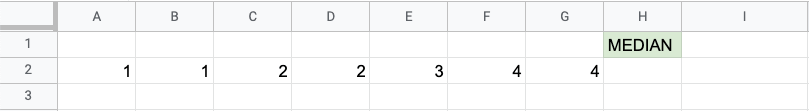MEDIAN function, step by step:

1. Select a cell `H2`
2. Type `=MEDIAN`
3. Click the MEDIAN command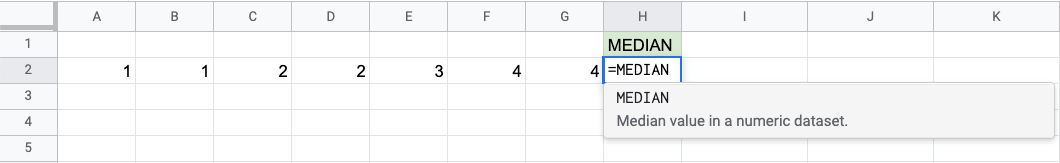1. Specify the range `A2:G2`
2. Hit enter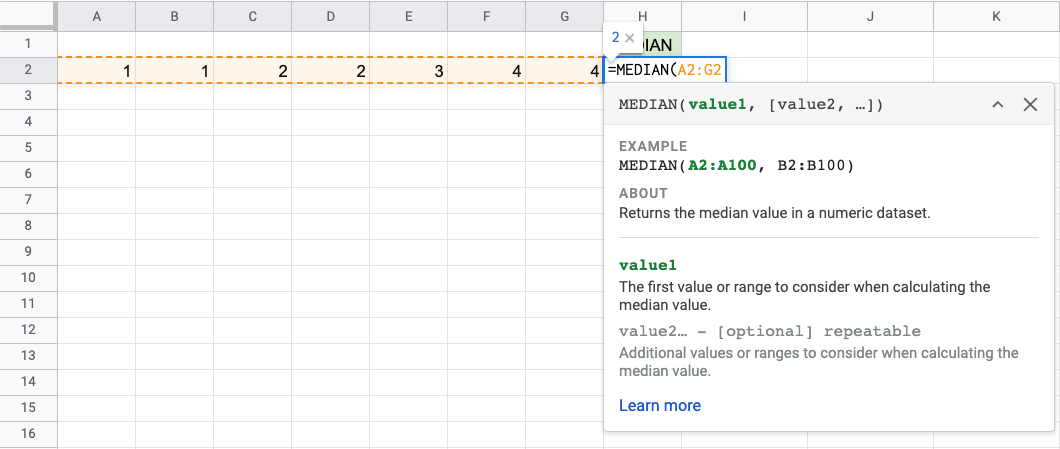Now, the function returns the median: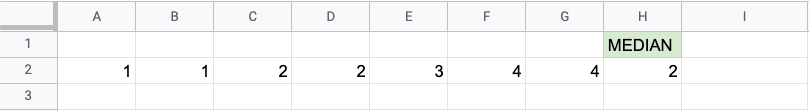Great! You successfully found the median using the `=MEDIAN` function.

Note that it found the value in the middle of the data, which is cell `D2`, the one that is marked red: